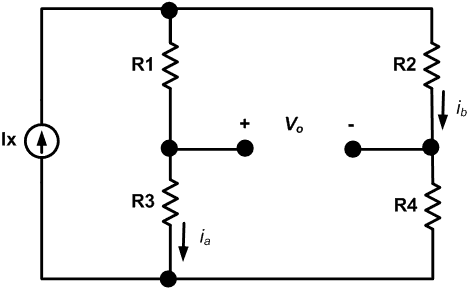# Determine currents ib and ia

• Cisneros778

## Homework StatementDetermine ib and ia

Ix = 0.4 mA, R1 = 15k ohm, R2 = 19k ohm, R3 = 23k ohm, R4 = 15k ohm

V=IR

## The Attempt at a Solution

I am assuming there are 4 unknown currents in this diagram.
Ix splits into ib and i1.

0.4mA - ib - i1 = 0
i1 - i2 = ia

R2*ib - Vo + R1*(0.4mA - ib) = 0

R3*ia + R4*(0.4mA - ia) = 0

I am confused about the independent current source. In some problems, I have seen it carry a voltage. So if I include a voltage in my analysis for that loop I will have another unknown.

Vx(0.4mA) + R1(0.4mA - ib) + R3(ia) = 0

Last edited:
Hint: Vx can be calculated from Ix and the series/parallel combination of the resistors. Then Ia and Ib are easily calculated.

Thank you, that makes sense